#### You may also like### Cubes

How many faces can you see when you arrange these three cubes in different ways?### Bracelets

Investigate the different shaped bracelets you could make from 18 different spherical beads. How do they compare if you use 24 beads?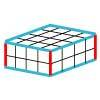### Making Cuboids

Let's say you can only use two different lengths - 2 units and 4 units. Using just these 2 lengths as the edges how many different cuboids can you make?

# Treasure Hunt

##### Age 7 to 14 Challenge Level:

Nathan from Frederick Irwin Anglican School in Australia and Phoebe from St Mary's Church of England Primary School Woodbridge in the UK described how they got closer to the treasure. Nathan wrote:
Starting in the centre square of 6, 6 then you have a number of steps you are away and you have all of the different sides to the treasure from there move 2 steps away from the centre in any direction this will then give you another number if it is more you have moved away and if it is less than you are closer if you are closer move the amount said froward if you are still not on the treasure then move 3 either left or right.

Matilda and Bethany from Frederick Irwin Anglican School, William from Kirkby Malzeard C of E Primary School in the UK and Keira, Lydia and Kitch from Hymers College in England and Kitch described another method for finding the treaure. Lydia wrote:
In this challenge, my strategy was to start in the middle because that would be the most logical place to start as it has the most coordinates directly around it. Then I went to a place that was the said number of steps away from the already chosen coordinate and then when the next amount of steps was shown it had to be the first shown amount of steps away from the first set of coordinates and the second amount of steps away from the second coordinates. I kept on doing this so that I soon found the treasure.

But make sure that those co-ordinates are also [the right number of] spaces away from your first co-ordinates because if they are not it couldn’t possibly be correct.

Srikar from Hymers College searched by choosing strategic points:
First choose (6,6) because it is in the middle of the board and you can work around it easily.
Then choose (3,3) and (9,9) because they are equally between (0,0) and (6,6) or (12,12) and (6,6). This will help by lowering [the number of] possibilities,.
Next find the two or so possibilities for the coordinates (using the mouse to make dots to help).
Finally test the possibilities to find the correct coordinates.

4/3C class from Strathfield North in Australia described their strategy:
Corner the number. So start in the middle and then pick a number in a corner. Then you work your way closer towards it.

Ruhara from St Brigid's Kyogle, NSW in Australia used a similar idea:
When I start, the first coordinate I choose is the center coordinate (6,6).
Then, since I am in the middle of the sand block I can easily divide the block into four squares. After splitting the board, I choose one of the squares randomly and type in the coordinate that is the center of the chosen square. When it gives me the number of steps to get to the treasure I can figure out if the treasure is inside that square or not. If I have to, I can repeat the strategy for every square.

Alex from Elm Park Primary School in New Zealand used the same idea to split the board into "rings":
A strategy I found to work well is where you go for the center. Going for the center shows what outer ring the treasure is in. After that you do that you then go for corners as they will show the most. After you choose that, you can then see how far it is, then you will either need to try with another corner, or if it is close then you can choose one of the two spots leading to it from how far it tells you.

Nrich Live Club from St Michael's International School, Kobe in Japan, Daisy and Izzy from Thomas Eaton Primary Academy in England, Lucia from Willowbank in New Zealand and Andrew from TFS in Canada used an idea similar to Jenna's and Alex's idea, of rings the same distance from the original point. Here is Nrich Live Club's work:
You can start anywhere on the map. We started in the middle so that you cover the whole map. So use the coordinates (6,6).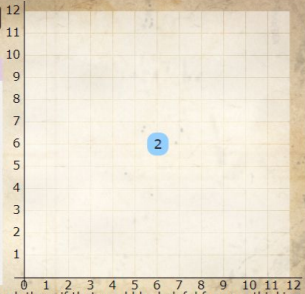Mark all the possible coordinates using the number of steps.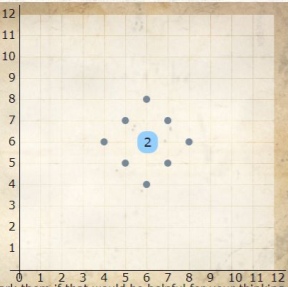Try one of the coordinates. We tried (6,8).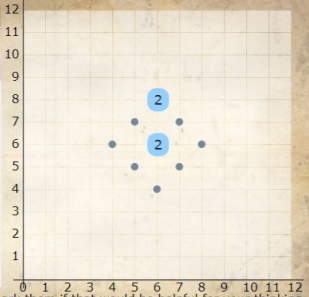We can now get rid of the coordinates which are no longer possible. For example, it cannot be (6,4) because that is 4 steps away from (6,8)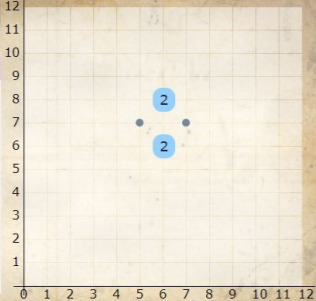Let's try (5,7). We found the treasure!

Lucia used the "rings" in a slightly different way:
First of all I select a place eg: (5,5). Next I plan all the possibilties on with the circles, you can do the by drawing a circle around the number. Next you select another number and you work out the possibilty of that. Make sure to draw EVERY possibilty. Now there will be two dots that will meet up. that will be where the treasure lies! So... enjoy your treasure! ;)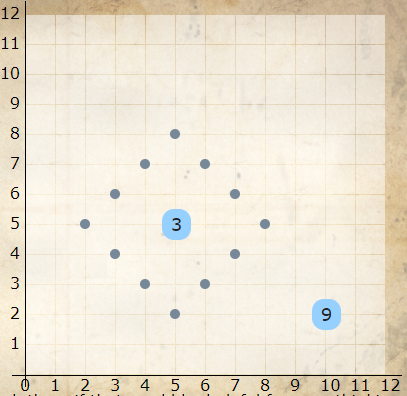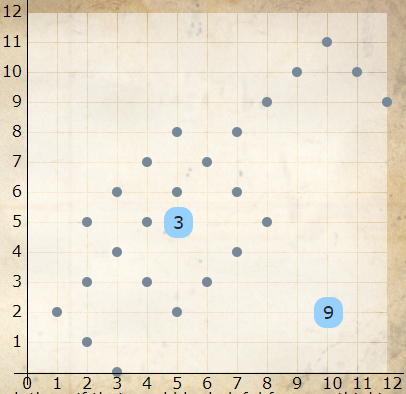Andrew used a different starting point to reduce the number of possibilities on both "rings":
Since guesses always create a diamond shape of points around them, placing a guess in one of the four corners will only leave us one edge of the diamond to look at. This equates to a single diagonal line passing through the grid.
Since we know that the treasure has to fall on this specific line, we can make a guess on one of those points and use the distance value to locate the exact point. Placing our guess in the centre of this line won’t work, as a distance value of 2 could mean that the point lies above or below the guess. However, placing your guess at the end of the line (where the line hits the edge of the grid) gives each possible point a unique distance value, which allows us to find the treasure.
Here’s an example game: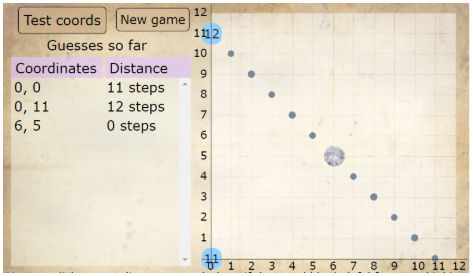With this method it’s possible to consistently find the treasure with fewer than 4 guesses.

Level 2.
This problem adds an extra step to the previous problem by randomly shifting the point of origin to another location on the grid. This doesn’t add too much extra work though, as it only requires reframing the problem as if the origin was in the corner. We progress through this problem the same way as level 1, starting in the bottom left corner, moving to the point where the line hits the edge of the grid then finally locating the treasure point.
With this method it’s possible to consistently find the treasure with fewer than 4 guesses.

Level 3.
Level 3 ramps up the complexity by restricting the guessing area to a pink region. This pink region can be in one of three possible orientations. Since two of the orientations are mirror images of each other, they will be treated the same and this level will be split into two cases: diagonal & straight.
a) straight region
Since the corners aren’t accessible due to the new limitations, placing your guess on the edge of the grid eliminates the most possibilities, leaving us with only two of the edges of the diamond pattern.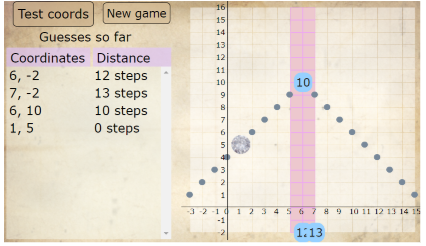My next step was to try and differentiate between the two sections of the diamond. I wanted a way to tell which side the treasure was on. Left or right? We can determine this by guessing the point one unit to the left or right of our original guess. If the point is in that direction, the distance will have lessened as we have moved closer. To avoid confusion, let’s say that we will always choose the [middle of the] bottom edge for the first guess and then move one unit to the right :
Let $d_1$ be the distance of the treasure from the first guess.
Let $d_2$ be the distance of the treasure from the second guess.
If $d_1 - d_2 = 1$, the point is to the right of the first guess.
If $d_1 - d_2 = -1$, the point is to the left or above of the first guess.
This distinction between left and right allows us to eliminate half of the possible locations, leaving us with one line of locations.

Our second last guess will happen at the point on the line with the height of the highest point on the line. That might sound a bit complicated, so let’s deconstruct it. Since our first guess took place in the bottom centre of this line, the highest point on the remaining line is either on the centre line (if $d_1 \le 18$) or is on the top edge of the grid (if $d1 \gt 18$). Placing our guess on the centre line at the height of the highest point [at $(8,d_1),$ if the origin was at the bottom left] ensures that in both cases each possible location has a unique distance value, which means we can place our next guess on the treasure!

Here’s another example straight region game with the initial distance greater than 18 :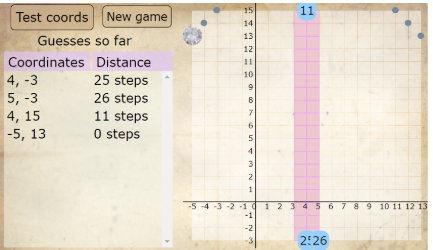With this method it’s possible to consistently find the treasure with fewer than 5 guesses.

b) diagonal region
In the case of the diagonal region, the corners are available and are used to their full potential, leaving us with a single line of possible locations.
The next step is to guess a point on the line that intersects the pink region. To keep results consistent, pick the right-most point intersecting the pink region. This guess will yield a distance that should narrow down the line to at most two possibilities. To distinguish the two points, guess the point one unit to the left of your second guess :
Let $d_2$ be the distance of the treasure from the second guess.
Let $d_3$ be the distance of the treasure from the third guess.
If $d_2 - d_3 = 1$, the point is to the left of the second guess.
If $d_2 - d_3 = -1$, the point is to the right of the second guess.

Place your final guess on the point singled out by your last three guesses.

Here’s an example diagonal region game :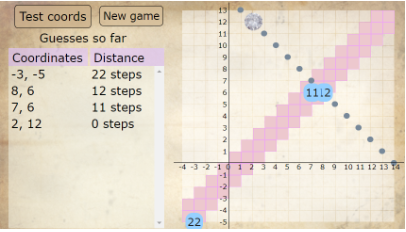With this method it’s possible to consistently find the treasure with fewer than 5 guesses.